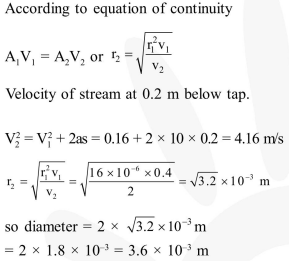Deepak Scored 45->99%ile with Bounce Back Crack Course. You can do it too!

# Water is flowing continuously

Question:

Water is flowing continuously from a tap having an internal diameter $8 \times 10^{-3} \mathrm{~m}$. The water velocity as it leaves the tap is $0.4 \mathrm{~ms}^{-1}$. The diameter of the water stream at a distance $2 \times 10^{-1} \mathrm{~m}$ below the tap is close to :-

1. $9.6 \times 10^{-3} \mathrm{~m}$

2. $3.6 \times 10^{-3} \mathrm{~m}$

3. $5.0 \times 10^{-3} \mathrm{~m}$

4. $7.5 \times 10^{-3} \mathrm{~m}$

Correct Option: , 2

Solution: